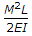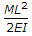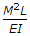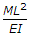# Civil Engineering - Strength of Materials

### Exercise :: Strength of Materials - Section 1

46.

The radius of gyration of a rectangular section is not proportional to

 A. square root of the moment of inertia B. square root of the inverse of the area C. square root of the moment of inertia divided by area of the section D. none of these.

Explanation:

No answer description available for this question. Let us discuss.

47.

A reinforced concrete beam is assumed to be made of

 A. homogeneous material B. heterogeneous material C. isotropic material D. none of these.

Explanation:

No answer description available for this question. Let us discuss.

48.

If the rivets in adjacent rows are staggered and outermost row has only one rivet, the arrangement of the rivets, is called

 A. chain riveting B. zig-zag riveting C. diamond riveting D. none of these.

Explanation:

No answer description available for this question. Let us discuss.

49.

The energy stored in a beam of length L subjected to a constant B.M. is

 A.B.C.D.Explanation:

No answer description available for this question. Let us discuss.

50.

The force in DB of the truss shown in below figure isA. 3 W compression B. W tension C. 2 W compression D. 5 W tension E. 4 W tension.